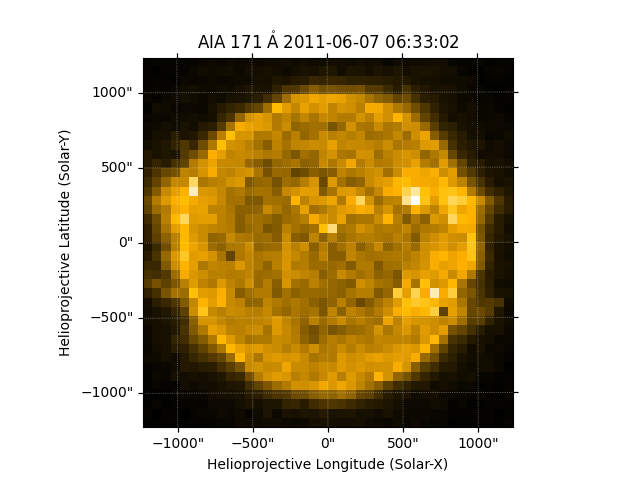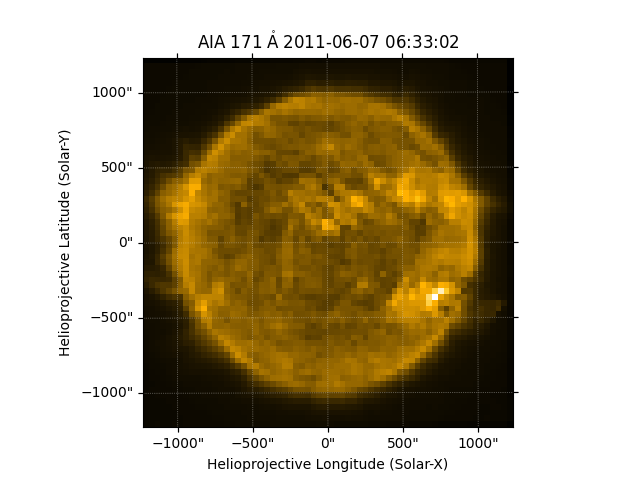# Resampling Maps¶

How to resample a map using the resample method, which implements interpolation, or using superpixels, which combines pixels.

```import matplotlib.pyplot as plt

import astropy.units as u

import sunpy.map
import sunpy.data.sample
```

```aia_map = sunpy.map.Map(sunpy.data.sample.AIA_171_IMAGE)
```

To reduce the angular resolution of the map you can use the `resample` method, specifying the new dimensions in pixels. By default, this method uses linear interpolation but this can be changed with the `method` argument (‘neighbor’, ‘nearest’, ‘linear’ or ‘spline’)

```new_dimensions = [40, 40] * u.pixel
aia_resampled_map = aia_map.resample(new_dimensions)
```

Let’s plot the result.

```ax = plt.subplot(projection=aia_resampled_map)
aia_resampled_map.plot()
plt.show()
```Another way to resample is by using the `superpixel` method. This can be used to increase the signal to noise ratio by reducing the resolution of the image by combining pixels. This means that the new dimension must divide the original size exactly. For example you can reduce the AIA map resolution by a factor of 16.

```new_dimensions = u.Quantity(aia_map.dimensions) / 16
aia_superpixel_map = aia_map.superpixel(new_dimensions)
```

Let’s plot the result.

```ax = plt.subplot(projection=aia_superpixel_map)
aia_superpixel_map.plot()
plt.show()
```Total running time of the script: ( 0 minutes 0.825 seconds)

Gallery generated by Sphinx-Gallery